Formatted question description: https://leetcode.ca/all/1914.html

# 1914. Cyclically Rotating a Grid

Medium

## Description

You are given an m x n integer matrix grid, where m and n are both even integers, and an integer k.

The matrix is composed of several layers, which is shown in the below image, where each color is its own layer: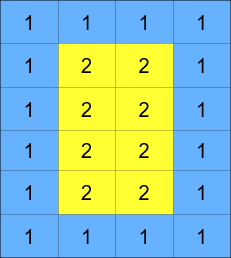A cyclic rotation of the matrix is done by cyclically rotating each layer in the matrix. To cyclically rotate a layer once, each element in the layer will take the place of the adjacent element in the counter-clockwise direction. An example rotation is shown below: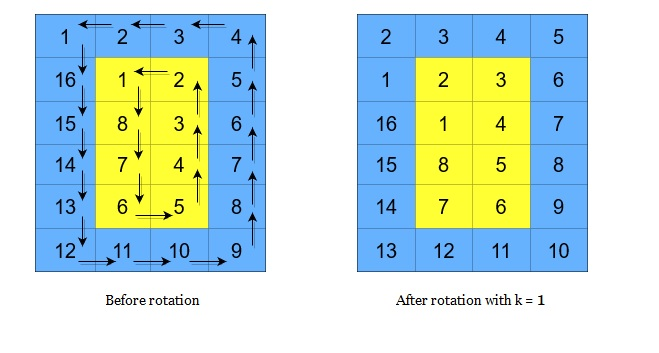Return the matrix after applying k cyclic rotations to it.

Example 1: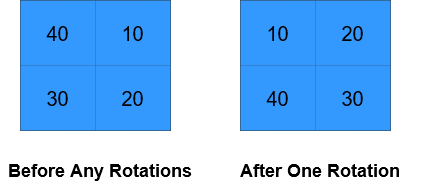Input: grid = [[40,10],[30,20]], k = 1

Output: [[10,20],[40,30]]

Explanation: The figures above represent the grid at every state.

Example 2: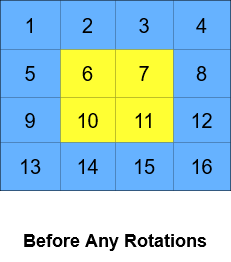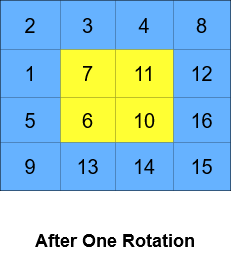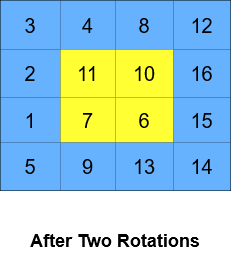Input: grid = [[1,2,3,4],[5,6,7,8],[9,10,11,12],[13,14,15,16]], k = 2

Output: [[3,4,8,12],[2,11,10,16],[1,7,6,15],[5,9,13,14]]

Explanation: The figures above represent the grid at every state.

Constraints:

• m == grid.length
• n == grid[i].length
• 2 <= m, n <= 50
• Both m and n are even integers.
• 1 <= grid[i][j] <= 5000
• 1 <= k <= 10^9

## Solution

First, get the spiral order of all the elements in grid. Next, split the elements into different layers, rotate the elements in each layer, and update the rotated spiral order. Finally, put the elements in the rotated spiral order into the grid.

To split the elements into different layers, the first layer has (m + n - 2) * 2 elements, and for the remaining layers, the number of elements in each layer will be subtracted by 8. When there are no remaining elements in the spiral order, all elements are visited.

class Solution {
public int[][] rotateGrid(int[][] grid, int k) {
int m = grid.length, n = grid.length;
List<Integer> order = spiralOrder(grid);
List<Integer> rotatedOrder = new ArrayList<Integer>();
int startIndex = 0;
int layerSize = (m + n - 2) * 2;
int total = m * n;
while (startIndex < total) {
List<Integer> layer = order.subList(startIndex, startIndex + layerSize);
int curIndex = k % layerSize;
for (int i = 0; i < layerSize; i++) {
curIndex = (curIndex + 1) % layerSize;
}
startIndex += layerSize;
layerSize -= 8;
}
return generateGrid(m, n, rotatedOrder);
}

public List<Integer> spiralOrder(int[][] grid) {
List<Integer> order = new ArrayList<Integer>();
int m = grid.length, n = grid.length;
int left = 0, right = n - 1, top = 0, bottom = m - 1;
while (left <= right && top <= bottom) {
for (int column = left; column <= right; column++)
for (int row = top + 1; row <= bottom; row++)
if (left < right && top < bottom) {
for (int column = right - 1; column > left; column--)
for (int row = bottom; row > top; row--)
}
left++;
right--;
top++;
bottom--;
}
return order;
}

public int[][] generateGrid(int m, int n, List<Integer> order) {
int[][] grid = new int[m][n];
int row = 0, column = 0;
int[][] directions = { {0, 1}, {1, 0}, {0, -1}, {-1, 0} };
int directionIndex = 0;
int size = order.size();
for (int i = 0; i < size; i++) {
grid[row][column] = order.get(i);
int nextRow = row + directions[directionIndex], nextColumn = column + directions[directionIndex];
if (nextRow < 0 || nextRow >= m || nextColumn < 0 || nextColumn >= n || grid[nextRow][nextColumn] != 0)
directionIndex = (directionIndex + 1) % 4;
row = row + directions[directionIndex];
column = column + directions[directionIndex];
}
return grid;
}
}